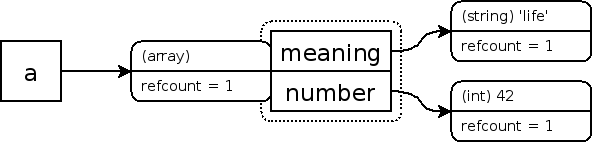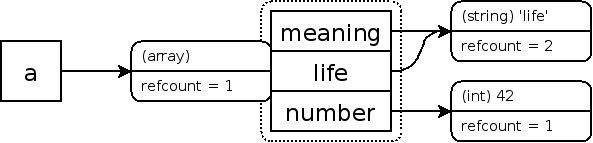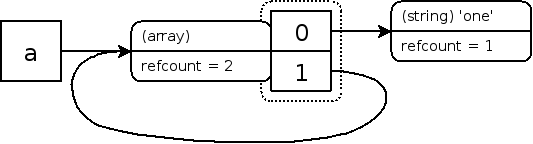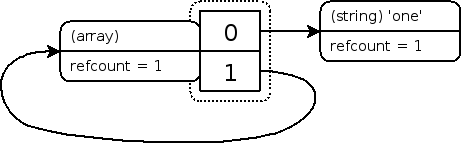一，php变量

php变量分变量名符号和变量内容，变量符号存在符号表中，而变量内容存储在zval变量容器，具体介绍：

1，zval结构

typedef union _zvalue_value {
long lval;                  /* long value */
double dval;                /* double value */
struct {
char *val;
int len;
} str;
HashTable *ht;              /* hash table value */
zend_object_value obj;
zend_ast *ast;
} zvalue_value;

struct _zval_struct {
zvalue_value value;     /*描述变量的值*/
zend_uint refcount__gc;
zend_uchar type;        /*描述变量的类型*/
zend_uchar is_ref__gc;
};

_zval_strust包含typevalue，另外包含两个字节的额外信息，is_ref：bool值，标示这个变量是否是属于引用集合(reference set)，php引擎通过is_ref才能把普通变量和引用变量区分开来。refcount：标示指向这个zval变量容器的变量(也称符号即symbol)个数，用来优化内存使用。

<?php

\$a = "new string";             // 生成一个新的zval容器

xdebug_debug_zval('a'); //结果：a: (refcount=1, is_ref=0),string 'new string' (length=11)

\$c = \$b = \$a;                  // 把一个变量赋值给另一变量，增加一个引用计数

xdebug_debug_zval('a'); //结果：a: (refcount=3, is_ref=0),string 'new string' (length=11)

\$b = '123';

xdebug_debug_zval('a'); //结果：a: (refcount=2, is_ref=0),string 'new string' (length=11)

unset(\$c);                   //变量调用了函数 unset()时，”refcount“就会减1

xdebug_debug_zval('a'); //结果：a: (refcount=1, is_ref=0),string 'new string' (length=11)

\$d = &\$a;

xdebug_debug_zval('a'); //结果：a: (refcount=2, is_ref=1),string 'new string' (length=11)

unset(\$a);                    //包含类型和值的这个变量容器就会从内存中删除。

xdebug_debug_zval('a'); //结果：a: no such symbol

?>

b变量=a，同一个变量容器被变量a和变量b关联，php不会复制已生成的变量容器，refcount变成2，unset变量时，refcount减1、当任何关联到某个变量容器的变量离开它的作用域(比如：函数执行结束)也会减1，当refcount变成0时就被销毁。

array和object符合类型，与普通类型不同，符合类型把成员和属性存在自己的符号表，会有多个zval变量容器

Example #2

<?php

\$a = array( 'meaning' => 'life', 'number' => 42 );    //Creating a array zval

xdebug_debug_zval( 'a' );

/* 结果：
a:(refcount=1, is_ref=0),
array (size=2)
'meaning' => (refcount=1, is_ref=0),string 'life' (length=4)
'number' => (refcount=1, is_ref=0),int 42
*/

?>Example #3 添加一个已经存在的元素到数组中

<?php

\$a = array( 'meaning' => 'life', 'number' => 42 );

\$a['life'] = \$a['meaning'];

xdebug_debug_zval( 'a' );

/*结果：
a:
(refcount=1, is_ref=0),
array (size=3)
'meaning' => (refcount=2, is_ref=0),string 'life' (length=4)
'number' => (refcount=1, is_ref=0),int 42
'life' => (refcount=2, is_ref=0),string 'life' (length=4)
*/

?>Example #4 从数组中删除一个元素

<?php

\$a = array( 'meaning' => 'life', 'number' => 42 );

\$a['life'] = \$a['meaning'];

unset( \$a['meaning'], \$a['number'] );

xdebug_debug_zval( 'a' );

/*结果：
a:
(refcount=1, is_ref=0),
array (size=1)
'life' => (refcount=1, is_ref=0),string 'life' (length=4)
*/

?>

Example #5 把数组作为一个元素添加到自己

<?php

\$a = array( 'one' );

\$a[] =& \$a;

xdebug_debug_zval( 'a' );

/*结果：
a:
(refcount=2, is_ref=1),
array (size=2)
0 => (refcount=1, is_ref=0),string 'one' (length=3)
'' => (refcount=2, is_ref=1),
&array<
*/

?>二，引用计数

变量的引用

PHP 的引用允许用两个变量来指向同一个内容。

<?php
\$a = " ABC " ;
\$b = &\$a ;
echo   \$a ; // 这里输出:ABC
echo   \$b ; // 这里输出:ABC
\$b = " EFG " ;
echo   \$a ; // 这里\$a的值变为EFG 所以输出EFG
echo   \$b ; // 这里输出EFG
?>

对象的引用(对象的赋值传递)

<?php
class fooclass{
public \$att ;
}
\$a = new fooclass();
\$b = \$a;
\$a->att = 1;
\$b->att = 2;
echo 'a obj:',(\$a->att),'<br>';
echo 'b obj:',(\$b->att);
/**
* 结果：
* a obj:2
b obj:2
*/

PHP5中默认就是通过引用来调用对象， 但有时你可能想建立一个对象的副本，并希望原来的对象的改变不影响到副本 . 为了这样的目的，PHP5定义了一个特殊的方法，称为__clone。

\$a = new foo fooclass(), 实际上\$a和new fooclass()的映射到不同对象实例，其中new fooclass产生的是一个匿名的fooclass对象实例 所以需要显式地使用\$a = & new fooclass()来进行引用赋值传递。

函数的引用传递（传址调用）

<?php
function  foo( & \$a ){
\$a = \$a + 100 ;
}
\$b = 1 ;
echo   \$b ; // 输出１
foo( \$b );// 这里\$b传递给函数的其实是\$b的变量内容所处的内存地址，通过在函数里改变\$a的值 就可以改变\$b的值了
echo   " <br> " ;
echo   \$b ; // 输出101

<?php
function  test( & \$b ){
\$b ++ ;
}
\$c = 0 ;
call_user_func_array ( 'test' , array ( & \$c ));
echo   \$c ;

函数的引用返回

<? php
function   & test()
{
static   \$b = 0 ; // 申明一个静态变量
\$b = \$b + 1 ;
echo   \$b ;
return   \$b ;
}
\$a = test(); // 这条语句会输出 \$b的值 为１
\$a = 5 ;
\$a = test(); // 这条语句会输出 \$b的值 为2
\$a =& test(); // 这条语句会输出 \$b的值 为3
\$a = 5 ;
\$a = test(); // 这条语句会输出 \$b的值 为6
?>

\$a=&test();
\$a=5;

& test()指出返回的是一个引用，而不是通常的一个拷贝。
\$a =& test()同样也指出 \$a 是作为引用的绑定，而不是通常的赋值。

[php] view plain copy print?

1. <?php
2. /**
3.  * This is the way how we  use  pointer to access variable inside the  class .
4.  */
5. class  talker{
6.      private   \$data   =   ' Hi ' ;
7.      public   function   &  get(){
8.          return   \$this -> data;
9.     }
10.     public   function  out(){
11.          echo   \$this -> data;
12.     }
13. }
14. \$aa   =   new  talker();
15. \$d   =   & \$aa -> get();
16. \$aa -> out();
17. \$d   =   ' How ' ;
18. \$aa -> out();
19. \$d   =   ' Are ' ;
20. \$aa -> out();
21. \$d   =   ' You ' ;
22. \$aa -> out();
23. //the output is  " Hi How Are You "

6 global 引用

[php] view plain copy print?

1. <?php
2.      \$var   =&   \$GLOBALS [ "var" ];
3. ?>

[php] view plain copy print?

1. <?php
2. global \$var;
3. \$var = "Example variable";
4. \$var_test =  &  \$GLOBALS [ 'var' ];
5. echo '\$var_test is set to ',\$var_test,'<br>'; //
6. \$var_test = 11;
7. unset(\$var_test);
8. echo '\$GLOBALS[var] is set to ',\$GLOBALS ['var'],'<br>';
9. /**
10.  * 输出结果：
11. \$var_test is set to Example variable
12. \$GLOBALS[var] is set to 11
13.  */

[php] view plain copy print?

1. \$var1 = "Example variable";
2. \$var2 = "";
3. function global_references(\$use_globals) {
4.     global \$var1, \$var2;
5.     if (!\$use_globals) {
6.         \$var2 =& \$var1; // visible only inside the function
7.     } else {
8.         \$GLOBALS["var2"] =& \$var1; // visible also in global context
9.     }
10. }
11. global_references(false);
12. echo "var2 is set to '\$var2'", '<br>'; // var2 is set to ''
13. global_references(true);
14. echo "var2 is set to '\$var2'"; // var2 is set to 'Example variable'

[php] view plain copy print?

1. \$var2 =& \$var1; // visible only inside the function

7 . 取消引用 unset与=null

[php] view plain copy print?

1. <?php
2.
3.      \$a   =   1 ;
4.      \$b   =&   \$a ;
5.      unset  ( \$a );
6.      echo \$b; //输出：1:

8 . \$this

9. PHP引用使用注意事项

\$a = array('a','c'...'n');
\$b = \$a;

[php] view plain copy print?

1. function printArray(&\$arr) //引用传递
2.  {
3.     print(count(\$arr));
4. }
5.  printArray(\$a);

[php] view plain copy print?

1. function printArray(\$arr)  //值传递
2.  {
3.      print(count(\$arr));
4.  }
5.  printArray(\$a);

[php] view plain copy print?

1. \$a = array(1,2,3);
2. \$b =\$a;
3. function printArray(\$arr)  //值传递
4. {
5.     print(count(\$arr));
6. }
7. \$s = microtime(true);
8. for(\$i=1;\$i<10000;\$i++){
9.     printArray(\$a);
10.  }
11. \$e = microtime(true);
12. echo '=----------------------------';
13. echo \$e-\$s;//0.031868934631348 s

[php] view plain copy print?

1. \$a = array(1,2,3);
2. \$b = \$a;
3. function printArray(&\$arr)  //引用传递
4. {
5.     print(count(\$arr));
6. }
7. \$s = microtime(true);
8. for(\$i=1;\$i<10000;\$i++){
9.     printArray(\$a);
10.  }
11. \$e = microtime(true);
12. echo '=----------------------------';
13. echo \$e-\$s;//0.047234058380127小疯子的逆时光

php的引用（就是在变量或者函数、对象等前面加上&符号）

php的引用（就是在变量或者函数、对象等前面加上&符号） 在PHP 中引用的意思是：不同的名字访问同一个变量内容. 与Ｃ语言中的指针是有差别的．Ｃ语言中的指针里面存储的是变量的内容在内存中...

2012/03/09
1K
0
php中引用&的真正理解

chenzy526
2017/05/26
0
0
PHP超全局变量、魔术变量和魔术方法

PHP在设计的时候已经预定义了9个超全局变量、8个魔术变量和13魔术方法，这些变量和函数可以在脚本的任何地方不用声明就可以使 用。 在PHP开发会频繁的使用这些变量和函数，这些变量和函数可以...

Weltch
2016/10/15
545
0
PHP的static与Java的static的对比

big_cat
2015/09/30
303
2
PHP超级全局变量、魔术变量和魔术函数

PHP在设计的时候已经预定义了9个超级全局变量、8个魔术变量和13魔术函数，这些变量和函数可以在脚本的任何地方不用声明就可以使用。 在PHP开发会频繁的使用这些变量和函数，这些变量和函数可...

a928154159
2018/02/22
0
0

PostgreSQL 11.3 locking

rudi

5
0
Mybatis Plus sql注入器

11
1

4
0

9
0

81
0